# Introduction to Python - Lesson One

Posted on 21-05-19 in Turtle Exercises

##### This post is part 1 of the Introduction to Programming" series
Introduction to Python - Lesson One

Introduction to programming using Python and Turtle

Introduction to Python - Lesson Two

Continuing our introduction to Python using Turtle

Introduction to Python - Lesson Three

Looking at loops and conditionals in more detail

Introduction to Python - Lesson Four

Further exploration of Turtle and an introduction to colour

Introduction to Python using Turtle : A Review

A brief review of the first part of this Turtle course

1. The importance of typing exactly
2. Drawing in Python using Turtle
3. Why we use variables and loops

## Getting Started

First off, open up IDLE! This is the editor we will be using for writing Python. Python is a popular programming language used all over the world.

IDLE has two modes we can use :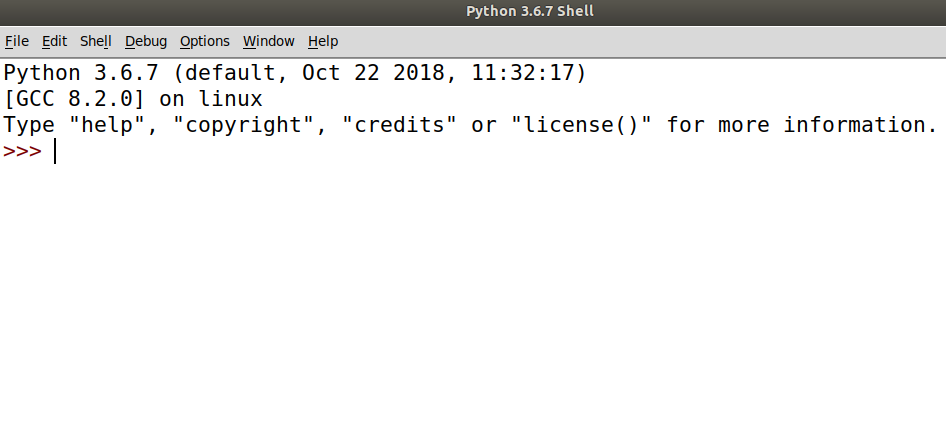Interactive Mode Here we can type commands the computer can understand and it will run immediately. This is good for playing around and learning how things work. It will not save your work.

If we click file we can create a new file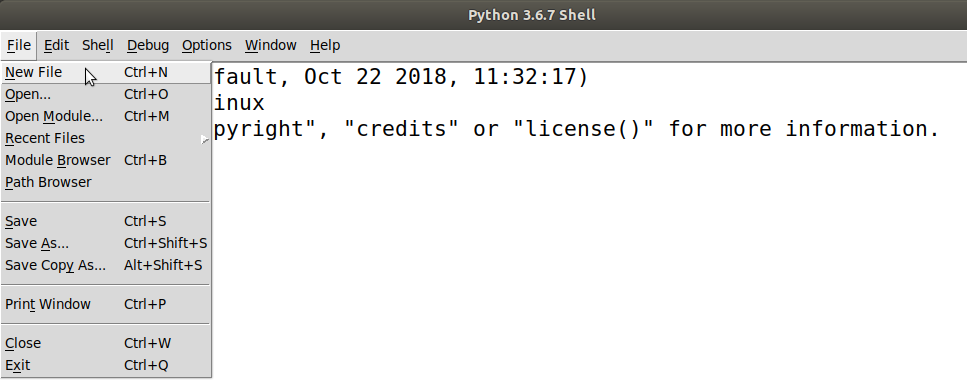This will let us write a program. Here the code is saved so we can refer back to it later!

## Drawing using Turtle

Let's begin by playing with a tool in Python called Turtle. This allows us to draw complicated patterns. We will limit ourselves initially to two commands :

• forward(units) - Moves the turtle forward units steps
• left(degrees) - Turns the turtle left by degrees degrees
• right(degrees) - Turns the turtle right by degrees degrees

if we're ever unsure about how something works in Python we can use help like so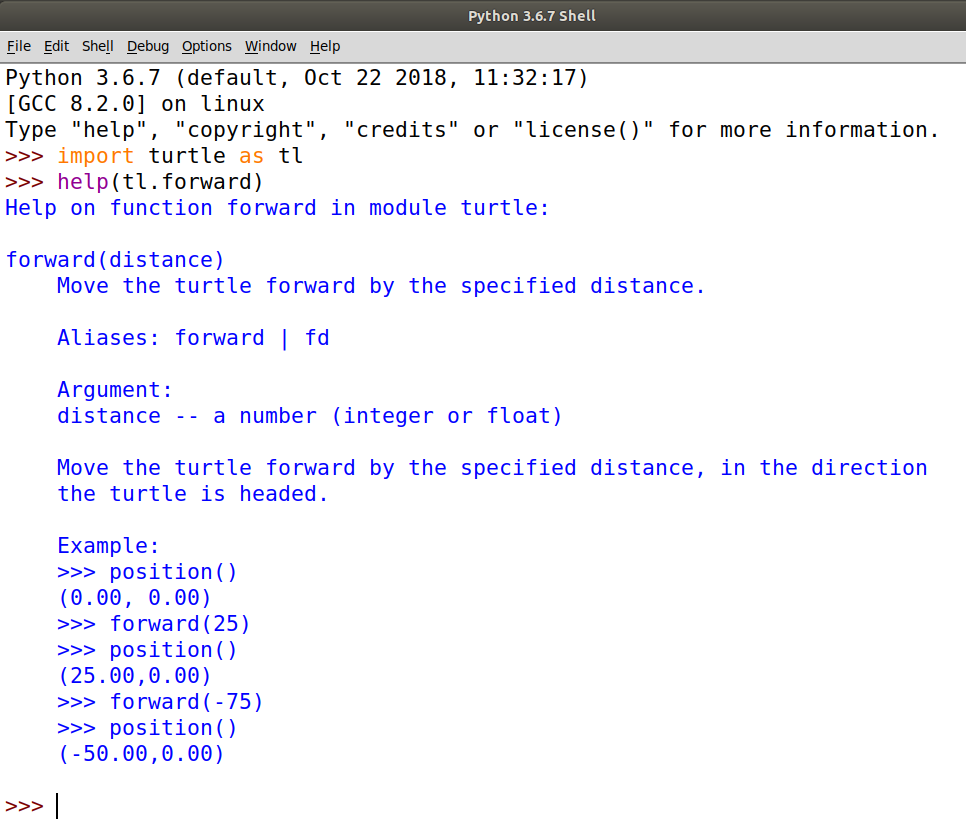Try getting the following code to work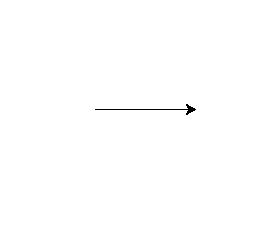```import turtle as tl
tl.forward(100)
```

and a square can be drawn like so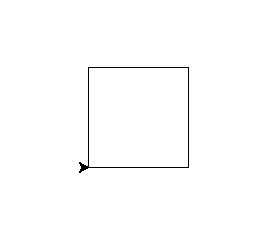```import turtle as tl
tl.forward(100)
tl.left(90)
tl.forward(100)
tl.left(90)
tl.forward(100)
tl.left(90)
tl.forward(100)
tl.left(90)
```

Exercise Set 1 1. Write the code to draw the following shapes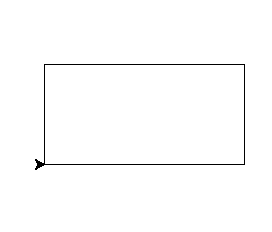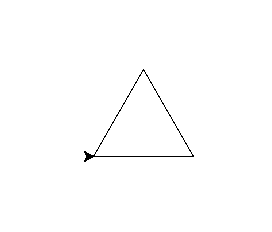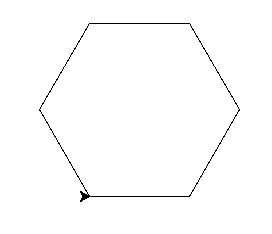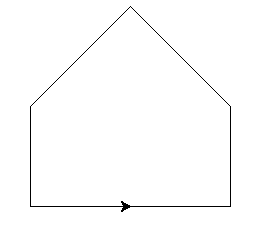In these cases we should notice how tedious it is to manually type the same lines of code over and over.

The most important principle to learn in programming is called DRY. This stands for DON'T REPEAT YOURSELF. This means that we need to find a way to stop typing the sames lines over and over. We want to avoid doing this, as it is hard to alter and easy to make mistakes!

For example, a square can be written as

```import turtle as tl

for _ in range(4):
tl.forward(100)
tl.left(90)
```

Here we have used a for loop. In this case it will repeat 4 times. We will explore how this works in more detail, in future classes. Remember you can use help(range) to find out more information!

Exercise Set 2

1. Rewrite the code for the previous shapes with loops
2. Draw the following spiral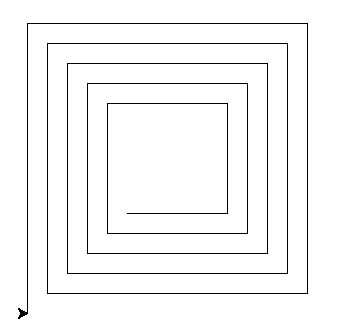Here we can see we need to use variables. These are objects with names that can hold numbers, text and other useful things.

```import turtle as tl

side = 100
angle = 90
sides = 4
loops = 5
increase_by = 10

for _ in range(loops*sides):
tl.forward(side)
tl.left(angle)
side = side + increase_by

tl.exitonclick()
```

Exercise Set 3

1. Alter just the variables to produce the following patterns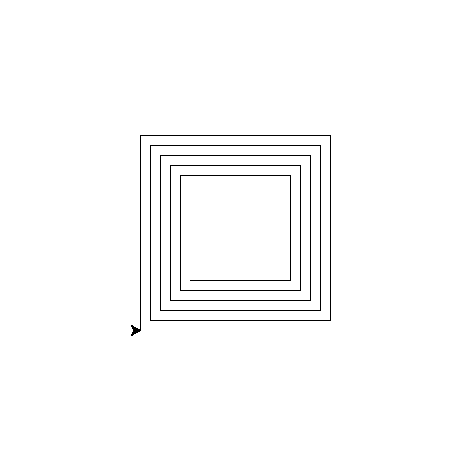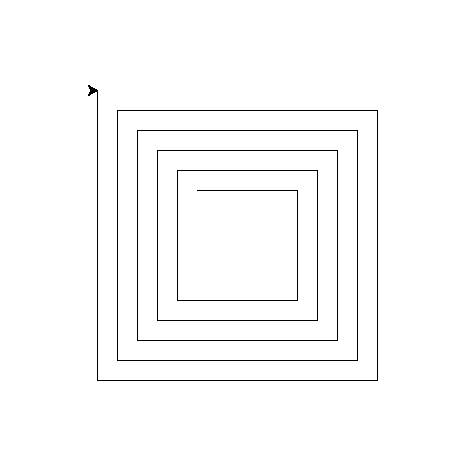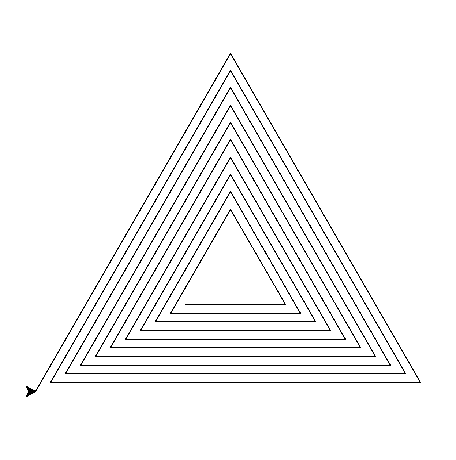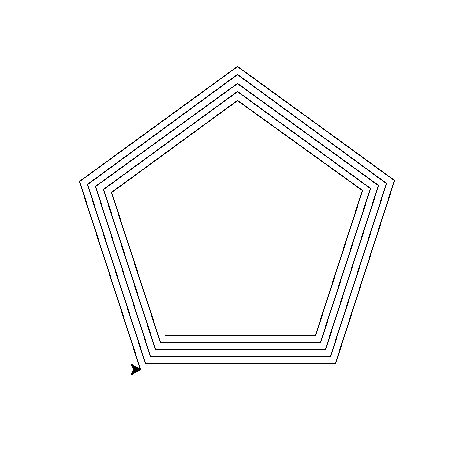1. Draw the following patterns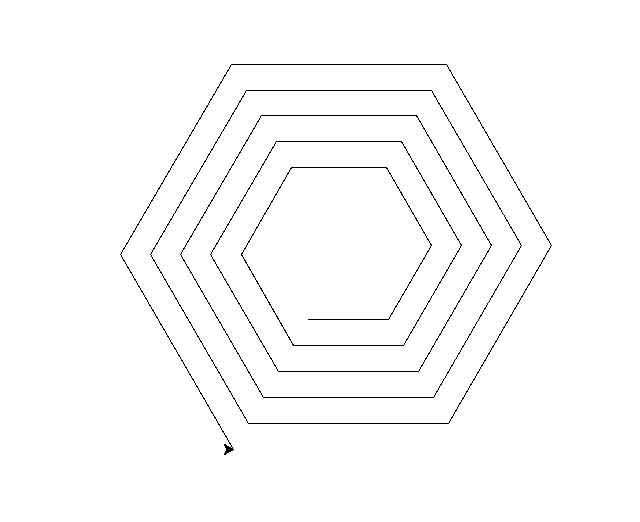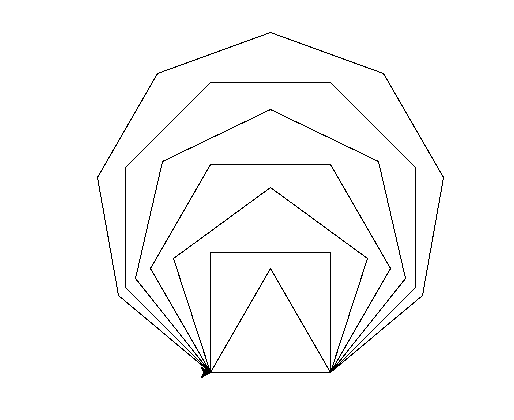## Conclusion

Today we have looked at using the left and forward commands in turtle with a for loop and variables.